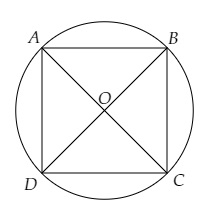# If a square is inscribed in a circle, find the ratio of the areas of the circle and the square.

Given:

A square is inscribed in a circle.

To do:

We have to find the ratio of the areas of the circle and the square.

Solution:

Let $r$ be the radius of the circle $s$ be the side of the square.This implies,

$AB = BC = CD = DA = s$

$AC$ and $BD$ are the diagonals of the square.

Diagonal $AC$ of the square $=$ Diameter of the circle

$\Rightarrow \sqrt{2} s=2 r$

$\Rightarrow s=\frac{2 r}{\sqrt{2}}$

Area of the circle $=\pi r^{2}$

Area of the square $=s^{2}$

The ratio of the areas of the circle and the square $=\frac{\pi r^{2}}{s^{2}}$

$=\frac{22 r^{2}}{7 \times(\frac{2 r}{\sqrt{2}})^{2}}$

$=\frac{22 r^{2}}{7 \times \frac{4 r^{2}}{2}}$

$=\frac{22 r^{2} \times 2}{7 \times 4 r^{2}}$

$=\frac{11}{7}$

The ratio of the areas of the circle and the square is $11:7$.

Updated on: 10-Oct-2022

49 Views